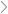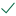Questions & AnswersStatistics and ProbabilityBigDeal Real Estate surveyed prices per square foot in the valley...

QuestionAnswered step-by-step

# BigDeal Real Estate surveyed prices per square foot in the valley...

BigDeal Real Estate surveyed prices per square foot in the valley and foothills of Hoke-a-mo, Utah. Based on BD's DATA, are prices per square foot equal at α = 0.01?

A) The critical value is 2.977 since this is a two-tail scenario. The test statistic is 3.207.  Since the test statistic > the critical value, the test statistic does lie in the area of rejection.  Reject the null hypothesis.  The prices per square foot are not equal at alpha = .01

B) The critical value is 2.977 since this is a two-tail scenario. The test statistic is 1.936.  Since the test statistic < the critical value, the test statistic does not lie in the area of rejection.  Do not reject the null hypothesis.  The prices per square foot are equal at alpha = .01

C) The critical value is 2.977 since this is a two-tail scenario. The test statistic is 1.513.  Since the test statistic < the critical value, the test statistic does not lie in the area of rejection.  Do not reject the null hypothesis.  The prices per square foot are equal at alpha = .01

D) The critical value is 2.977 since this is a two-tail scenario. The test statistic is 2.239.  Since the test statistic < the critical value, the test statistic does not lie in the area of rejection.  Do not reject the null hypothesis.  The prices per square foot are equal at alpha = .01Solved by verified expert

sectetur adipiscing elit. Nam lacinia pulvinar tortor nec facilisis. Pellentesque dapibus efficitur laoreet. Nam risus ante, dapibus a molestie consequat, ultrices ac magna. Fusce dui lectus, congue vel laoreet ac, dictum vitae odio. Donec aliquet. Lorem ipsum dolor sit amet, consectetur adipiscing10,000 step-by-step explanations

<p>sectetur adipiscing eli</p>sectetur adipiscing elit. Nam lacinia pulvinar tortor nec facilisis. Pellentesque dapibus efficitur laoreet. Nam risus ante, dapibus a molestie consequat, ultrices ac magna. Fusce dui lectus, congue vel laoreet ac, dictum vitae odio. Donec aliquet. Lorem ipsum dolor sit amet, consectetur adipiscing

## Step-by-step explanation